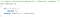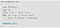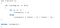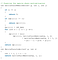# DYNAMIC PROGRAMMING

Dynamic programming (also known as DP) is a technique for solving a specific class of problems. The given problem can be divided into smaller sub-problems, and these smaller sub-problems are then divided into even smaller ones, and you may notice some over-lapping subproblems during this process. Furthermore, the optimal solutions to the subproblems contribute to the optimal solution of the given problem.

There are two ways

1. Memoization: Top-Down
2. Tabulation: Bottom-Up

Memoization: Solving the given problem by breaking it down and then return the solved answer.

Tabulation: Analyze the problem and see the order in which subproblems are solved and start solving from the trivial subproblem, up towards the given problem.

Divide and conquer is different from dynamic programing as in divide and conquer we divide the problem in non-overlapping subproblems and solve them independently.

There are two properties of the Dynamic Programming

1. Overlapping Subproblems
2. Optimal Substructure

# Overlapping Subproblems:

Dynamic Programming also combines the sub-problem like we use to do in divide and conquer. But the dynamic programming is used when the solution of the same subproblems is needed again and again. Dynamic programming, computed solutions to subproblems are stored in a table so that the same solution doesn’t have to be recomputed.Simple Recursive ProgramTabulated versionMemoized version

# Optimal Substructure:

A given problem has Optimal Substructure Property if an optimal solution of the given problem can be obtained by using optimal solutions of its subproblems.

For example, the Shortest Path problem has the following optimal substructure property: If a node x lies in the shortest path from a source node u to destination node v then the shortest path from u to v is a combination of the shortest path from u to x and shortest path from x to v. The standard All Pair Shortest Path algorithms like Floyd-Warshall and Bellman-Ford are typical examples of Dynamic Programming.

When solving a problem using dynamic programming, we have to follow three steps:

• Determine the recurrence relation that applies to said problem
• Initialize the memory/array/matrix’s starting values
• Make sure that when we make a “recursive call” (access the memoized solution of a subproblem) it’s always solved in advance

Following these rules, let’s take a look at some examples of algorithms that use dynamic programming.

# Applications of Dynamic Programming Approach

• Matrix Chain Multiplication
• Longest Common Subsequence
• Travelling Salesman Problem

# Matrix chain multiplication problem using Dynamic Programming :

We are covered a many of the real world problems.In our day to day life when we do making coin change, robotics world, aircraft, mathematical problems like Fibonacci sequence, simple matrix multiplication of more then two matrices and its multiplication possibility is many more so in that get the best and optimal solution. Now we can look about one problem that is matrix multiplication chain problem.

Suppose, We are given a sequence (chain) (A1, A2……An) of n matrices to be multiplied, and we wish to compute the product (A1A2…..An).We can evaluate the above expression using the standard algorithm for multiplying pairs of matrices as a subroutine once we have parenthesized it to resolve all ambiguities in how the matrices are multiplied together. Matrix multiplication is associative, and so all parenthesizations yield the same product. For example, if the chain of matrices is (A1, A2, A3, A4) then we can fully parenthesize the product (A1A2A3A4) in ﬁve distinct ways:

1:-(A1(A2(A3A4))) ,

2:-(A1((A2A3)A4)),

3:- ((A1A2)(A3A4)),

4:-((A1(A2A3))A4),

5:-(((A1A2)A3)A4) .

We can multiply two matrices A and B only if they are compatible. the number of columns of A must equal the number of rows of B. If A is a p x q matrix and B is a q x r matrix,the resulting matrix C is a p x r matrix. The time to compute C is dominated by the number of scalar multiplications is pqr. we shall express costs in terms of the number of scalar multiplications.

Note that in the matrix-chain multiplication problem, we are not actually multiplying matrices. Our goal is only to determine an order for multiplying matrices that has the lowest cost.

So problem is we can perform a many time of cost multiplication and repeatedly the calculation is performing. so this general method is very time consuming and tedious.So we can apply dynamic programming for solve this kind of problem.

# Dynamic Programming Approch to solve this problem:

1) Optimal Substructure:
A simple solution is to place parenthesis at all possible places, calculate the cost for each placement and return the minimum value. In a chain of matrices of size n, we can place the first set of parenthesis in n-1 ways. For example, if the given chain is of 4 matrices. let the chain be ABCD, then there are 3 ways to place first set of parenthesis outer side: (A)(BCD), (AB)(CD) and (ABC)(D). So when we place a set of parenthesis, we divide the problem into subproblems of smaller size. Therefore, the problem has optimal substructure property and can be easily solved using recursion.
Minimum number of multiplication needed to multiply a chain of size n = Minimum of all n-1 placements (these placements create subproblems of smaller size)

2) Overlapping Subproblems
Following is a recursive implementation that simply follows the above optimal substructure property.Memoised Approach

Time Complexity: O(n^3 )
Auxiliary Space: O(n^2)

# Conclusion

Dynamic programming is a tool that can save us a lot of computational time in exchange for a bigger space complexity, granted some of them only go halfway (a matrix is needed for memoization, but an ever-changing array is used).

This highly depends on the type of system you’re working on, if CPU time is precious, you opt for a memory-consuming solution, on the other hand, if your memory is limited, you opt for a more time-consuming solution for a better time/space complexity ratio.